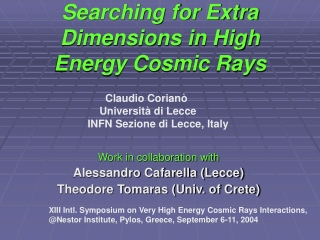Download PresentationSearching for Extra Dimensions in High Energy Cosmic Rays

# Searching for Extra Dimensions in High Energy Cosmic Rays - PowerPoint PPT PresentationDownload Presentation## Searching for Extra Dimensions in High Energy Cosmic Rays

- - - - - - - - - - - - - - - - - - - - - - - - - - - E N D - - - - - - - - - - - - - - - - - - - - - - - - - - -
##### Presentation Transcript

1. Searching for Extra Dimensions in High Energy Cosmic Rays Claudio Corianò Università di Lecce INFN Sezione di Lecce, Italy Work in collaboration with Alessandro Cafarella (Lecce) Theodore Tomaras (Univ. of Crete) XIII Intl. Symposium on Very High Energy Cosmic Rays Interactions, @Nestor Institute, Pylos, Greece, September 6-11, 2004

2. Theories with extra dimensions predict a gravity scale close to the electroweak scale, say 1 TeV, which can be accessed at future colliders (even the LHC). Even more so, in UHECR, we should be able to cross this (and the supersymmetry) scale by a large amount. Gravitational and Supersymmetric effects should appear at some stage in the dynamics of the extensive air showers. How? Can we look for specific signatures and strategies in order to get ready for possible surprises?

3. Extra Dimensional models are based on the idea that we live on a BRANE (a domain wall) immersed in a bigger space. String theories live in D=10= 9(space) +1(time) spacetime dimensions. ED models assume a spacetime structure in which p coordinates describe the brane and (9- p) are the remaining “extra” space coordinates. These extra coordinates are characterized by a compactification radius R which can be of a millimeter (the extra dimensional space is called: the bulk). Gravity can go into the bulk (ED) Matter stays on the brane. The Planck scale we are used to (MPlanck) is not the true scale for gravity.

4. There can be, additionally, “Kaluza Klein dimensions” Example: D=10 = 9 +1 = (3 +1) + (Nkk) + n Nkk= Kaluza-Klein dimensions D= 4 + n n= number of extra dimensions. We can have up to n=6 extra dimensions (Nkk=0) The scale of gravity is lowered (M *) << MPlanck Gravity becomes strong as soon as we reach M*, which is the true scale for gravity. It can be of the order of the electroweak scale (say M* = 1 TeV) . Gravitational effects which ordinarily occur for very large masses, say 1.5 times the solar mass, are now possible at an equivalent energy E > M*

5. RH = radius of the horizon (for the Sun is few km) Example: “squeeze the sun” within a sphere of about 3 km  you get a BH For the Earth is few cm For ED theories an horizon of 10-3 fm can form if the impact parameter of the collision is of this order. Very High Energy Neutrinos are the natural candidates for mini black hole formation. Factorization scales. In pp collisions it is required larger center-of-mass energy. For neutrino primaries The probability is larger (ν p collision) Giddings and Thomas, Nambu Gravitational schock-waves

6. As in top-down models also in BH decay there can be overlap with susy Effects in the fragmentation (C.C.); (C.C., A Faraggi) (Sarkar and Toldrà); (Berezinsky, Kachelriess et al.)

7. Various types of solutions Schwarzschild (static, uncharged) Reissner-Nordstrom (static, charged) Kerr (rotating) Boyer- Lindquist (rotating and charged) Large activity on Extra-D black holes in the last 4 years. Kanti and March-Russel Anchordoqui, Feng, Shapere Ahn, Ave, Cavaglià and Olinto Truly, we need angular momentum and the Kerr solution should be the starting point of the all analysis.

8. PHASES in BH DECAY 1) Balding Phase ( loose of hair, charge and other quantum numbers) 2) Spin down phase (from Kerr to Schwarzschild) 3) Schwarzschild phase (semiclassical Hawking description) 4) Planck phase (unknown) REMARKS: a) Multiplicities are computed only approximately. b) There are modifications of various types. For instance a generalized undetermination principle (GUP) can lower the multiplicites c) Issues regarding the presence of a chromosphere in BH decay (Heckler)

9. Multiplicities depend on the final phase of the black hole (DL= Dimopoulos and Landsberg) (N(n) Cavaglià, S. Das) entropy

10. We have studied the multiplicites and the lateral distributions of the air showers generated by an incoming high energy cosmic ray and mediated by the formation of an intermediate black hole The only way to attack this problem is to use the semiclassical Hawking Description, where a BH is viewed as a black body emitting thermal Blackbody radiation with a given characteristic temperature. Computed greybody factors (i.e. absorption/emission cross sections of black holes) analytically and numerically for most cases (with no angular momentum).

11. The BH decays into partons and leptons. The partons hadronize as soon as they cross the horizon. We use QCD fragmentation functions D(x, Q), evolved to the scale E/N

12. Uncorrelated decay of the BH into partons: multimonomial distribution p= decay probabilities We compute the total probability to produce a hadron h of a given energy E*h.

13. Energy is lost also in the bulk. These are gravitons which escape detection and the energy which is available for the Actual fragmentation is lower. Harris and Kanti

14. IF the ED scenario is correct, then we should see mini BH produced at hadron colliders and in cosmic rays. We have analized the lateral distributions and the multiplicities of cosmic rays events mediated by the presence of a resonance of this type. Larger multiplicities, wider spreading compared to ordinary events of comparablle characteristics. Open issues: gravitational emission. This requires the study of quasi-normal modes of gravitational perturbations (see Kokkotas’ review) (which is under investigation for the detection of gravitational waves from massive black holes )